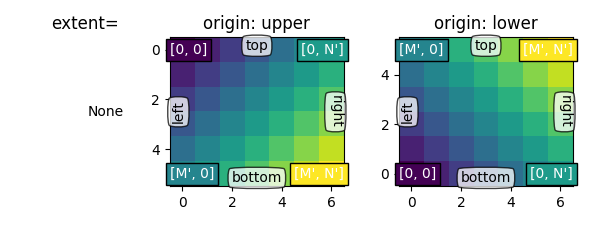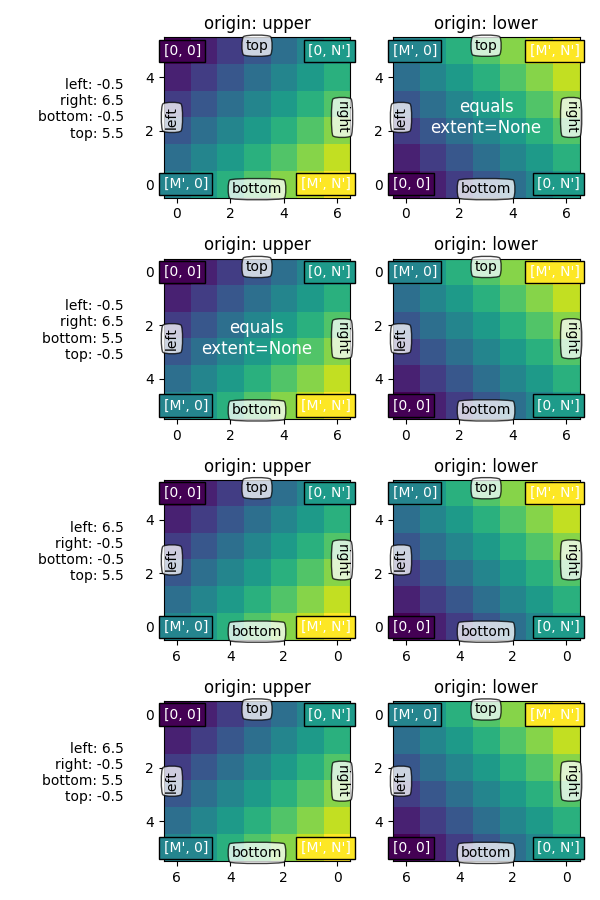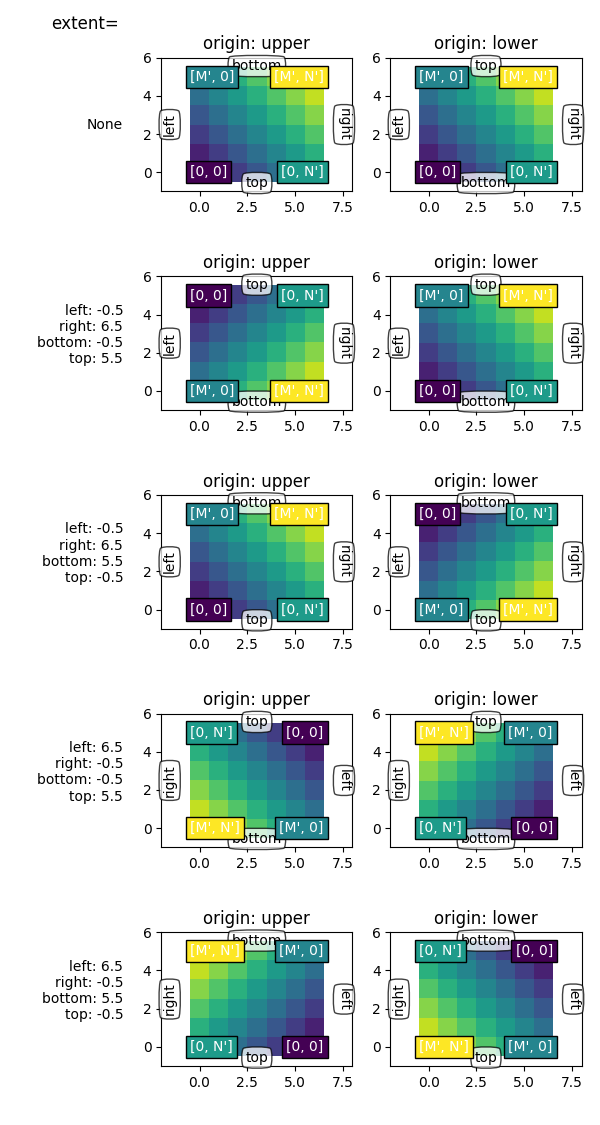# origin and extent in `imshow`¶

`imshow()` allows you to render an image (either a 2D array which will be color-mapped (based on norm and cmap) or and 3D RGB(A) array which will be used as-is) to a rectangular region in dataspace. The orientation of the image in the final rendering is controlled by the origin and extent kwargs (and attributes on the resulting `AxesImage` instance) and the data limits of the axes.

The extent kwarg controls the bounding box in data coordinates that the image will fill specified as `(left, right, bottom, top)` in data coordinates, the origin kwarg controls how the image fills that bounding box, and the orientation in the final rendered image is also affected by the axes limits.

Hint

Most of the code below is used for adding labels and informative text to the plots. The described effects of origin and extent can be seen in the plots without the need to follow all code details.

For a quick understanding, you may want to skip the code details below and directly continue with the discussion of the results.

```import numpy as np
import matplotlib.pyplot as plt
from matplotlib.gridspec import GridSpec

def index_to_coordinate(index, extent, origin):
"""Return the pixel center of an index."""
left, right, bottom, top = extent

hshift = 0.5 * np.sign(right - left)
left, right = left + hshift, right - hshift
vshift = 0.5 * np.sign(top - bottom)
bottom, top = bottom + vshift, top - vshift

if origin == 'upper':
bottom, top = top, bottom

return {
"[0, 0]": (left, bottom),
"[M', 0]": (left, top),
"[0, N']": (right, bottom),
"[M', N']": (right, top),
}[index]

def get_index_label_pos(index, extent, origin, inverted_xindex):
"""
Return the desired position and horizontal alignment of an index label.
"""
if extent is None:
extent = lookup_extent(origin)
left, right, bottom, top = extent
x, y = index_to_coordinate(index, extent, origin)

is_x0 = index[-2:] == "0]"
halign = 'left' if is_x0 ^ inverted_xindex else 'right'
hshift = 0.5 * np.sign(left - right)
x += hshift * (1 if is_x0 else -1)
return x, y, halign

def get_color(index, data, cmap):
"""Return the data color of an index."""
val = {
"[0, 0]": data[0, 0],
"[0, N']": data[0, -1],
"[M', 0]": data[-1, 0],
"[M', N']": data[-1, -1],
}[index]
return cmap(val / data.max())

def lookup_extent(origin):
"""Return extent for label positioning when not given explicitly."""
if origin == 'lower':
return (-0.5, 6.5, -0.5, 5.5)
else:
return (-0.5, 6.5, 5.5, -0.5)

def set_extent_None_text(ax):
ax.text(3, 2.5, 'equals\nextent=None', size='large',
ha='center', va='center', color='w')

def plot_imshow_with_labels(ax, data, extent, origin, xlim, ylim):
"""Actually run ``imshow()`` and add extent and index labels."""
im = ax.imshow(data, origin=origin, extent=extent)

# extent labels (left, right, bottom, top)
left, right, bottom, top = im.get_extent()
if xlim is None or top > bottom:
upper_string, lower_string = 'top', 'bottom'
else:
upper_string, lower_string = 'bottom', 'top'
if ylim is None or left < right:
port_string, starboard_string = 'left', 'right'
inverted_xindex = False
else:
port_string, starboard_string = 'right', 'left'
inverted_xindex = True
bbox_kwargs = {'fc': 'w', 'alpha': .75, 'boxstyle': "round4"}
ann_kwargs = {'xycoords': 'axes fraction',
'textcoords': 'offset points',
'bbox': bbox_kwargs}
ax.annotate(upper_string, xy=(.5, 1), xytext=(0, -1),
ha='center', va='top', **ann_kwargs)
ax.annotate(lower_string, xy=(.5, 0), xytext=(0, 1),
ha='center', va='bottom', **ann_kwargs)
ax.annotate(port_string, xy=(0, .5), xytext=(1, 0),
ha='left', va='center', rotation=90,
**ann_kwargs)
ax.annotate(starboard_string, xy=(1, .5), xytext=(-1, 0),
ha='right', va='center', rotation=-90,
**ann_kwargs)
ax.set_title('origin: {origin}'.format(origin=origin))

# index labels
for index in ["[0, 0]", "[0, N']", "[M', 0]", "[M', N']"]:
tx, ty, halign = get_index_label_pos(index, extent, origin,
inverted_xindex)
facecolor = get_color(index, data, im.get_cmap())
ax.text(tx, ty, index, color='white', ha=halign, va='center',
bbox={'boxstyle': 'square', 'facecolor': facecolor})
if xlim:
ax.set_xlim(*xlim)
if ylim:
ax.set_ylim(*ylim)

def generate_imshow_demo_grid(extents, xlim=None, ylim=None):
N = len(extents)
fig = plt.figure(tight_layout=True)
fig.set_size_inches(6, N * (11.25) / 5)
gs = GridSpec(N, 5, figure=fig)

columns = {'label': [fig.add_subplot(gs[j, 0]) for j in range(N)],
'upper': [fig.add_subplot(gs[j, 1:3]) for j in range(N)],
'lower': [fig.add_subplot(gs[j, 3:5]) for j in range(N)]}
x, y = np.ogrid[0:6, 0:7]
data = x + y

for origin in ['upper', 'lower']:
for ax, extent in zip(columns[origin], extents):
plot_imshow_with_labels(ax, data, extent, origin, xlim, ylim)

for ax, extent in zip(columns['label'], extents):
text_kwargs = {'ha': 'right',
'va': 'center',
'xycoords': 'axes fraction',
'xy': (1, .5)}
if extent is None:
ax.annotate('None', **text_kwargs)
ax.set_title('extent=')
else:
left, right, bottom, top = extent
text = ('left: {left:0.1f}\nright: {right:0.1f}\n' +
'bottom: {bottom:0.1f}\ntop: {top:0.1f}\n').format(
left=left, right=right, bottom=bottom, top=top)

ax.annotate(text, **text_kwargs)
ax.axis('off')
return columns
```

## Default extent¶

First, let’s have a look at the default `extent=None`

```generate_imshow_demo_grid(extents=[None])
```Generally, for an array of shape (M, N), the first index runs along the vertical, the second index runs along the horizontal. The pixel centers are at integer positions ranging from 0 to `N' = N - 1` horizontally and from 0 to `M' = M - 1` vertically. origin determines how to the data is filled in the bounding box.

For `origin='lower'`:

• [0, 0] is at (left, bottom)
• [M’, 0] is at (left, top)
• [0, N’] is at (right, bottom)
• [M’, N’] is at (right, top)

`origin='upper'` reverses the vertical axes direction and filling:

• [0, 0] is at (left, top)
• [M’, 0] is at (left, bottom)
• [0, N’] is at (right, top)
• [M’, N’] is at (right, bottom)

In summary, the position of the [0, 0] index as well as the extent are influenced by origin:

origin [0, 0] position extent
upper top left `(-0.5, numcols-0.5, numrows-0.5, -0.5)`
lower bottom left `(-0.5, numcols-0.5, -0.5, numrows-0.5)`

The default value of origin is set by `rcParams["image.origin"]` which defaults to `'upper'` to match the matrix indexing conventions in math and computer graphics image indexing conventions.

## Explicit extent¶

By setting extent we define the coordinates of the image area. The underlying image data is interpolated/resampled to fill that area.

If the axes is set to autoscale, then the view limits of the axes are set to match the extent which ensures that the coordinate set by `(left, bottom)` is at the bottom left of the axes! However, this may invert the axis so they do not increase in the ‘natural’ direction.

```extents = [(-0.5, 6.5, -0.5, 5.5),
(-0.5, 6.5, 5.5, -0.5),
(6.5, -0.5, -0.5, 5.5),
(6.5, -0.5, 5.5, -0.5)]

columns = generate_imshow_demo_grid(extents)
set_extent_None_text(columns['upper'])
set_extent_None_text(columns['lower'])
```## Explicit extent and axes limits¶

If we fix the axes limits by explicity setting `set_xlim` / `set_ylim`, we force a certain size and orientation of the axes. This can decouple the ‘left-right’ and ‘top-bottom’ sense of the image from the orientation on the screen.

In the example below we have chosen the limits slightly larger than the extent (note the white areas within the Axes).

While we keep the extents as in the examples before, the coordinate (0, 0) is now explicitly put at the bottom left and values increase to up and to the right (from the viewer point of view). We can see that:

• The coordinate `(left, bottom)` anchors the image which then fills the box going towards the `(right, top)` point in data space.
• The first column is always closest to the ‘left’.
• origin controls if the first row is closest to ‘top’ or ‘bottom’.
• The image may be inverted along either direction.
• The ‘left-right’ and ‘top-bottom’ sense of the image may be uncoupled from the orientation on the screen.
```generate_imshow_demo_grid(extents=[None] + extents,
xlim=(-2, 8), ylim=(-1, 6))
```Keywords: matplotlib code example, codex, python plot, pyplot Gallery generated by Sphinx-Gallery